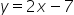Mathematics
Easy

Question

# A line is parallel to. The slope is ______.

## The correct answer is: 2

### Slope of the equation can be obtained by comapring with the general equationThe line is y=2x-7.The m represents a slope in y=mx+b.The slope of a parallel line will be the same.m=2

Hence slope is 2

### Related Questions to study#### With Turito Foundation.#### Get an Expert Advice From Turito.